# An easy way to remember Euclid GCD algorithm: laying tiles

I was a little embarrassed that I’ve never came across Euclid’s GCD algorithm until years after I graduated with Math and Engineering degrees. The descriptions I found online (especially Wikipedia) are convoluted and confusing, and the mechanical description of the algorithm does not shine light to any intuition. Then there comes proofs and abstract algebra properties that even after I followed the reasoning, I’d soon forget after I stop looking at it in a few weeks.

Just by staring at one simple description of the algorithm:

The classic algorithm for computing the GCD, known as Euclid’s algorithm, goes as follows: Let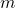and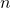be variables containing the two numbers, divideby. Save the divisor in, and save the remainder in. Ifis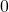, then stop:contains the GCD. Otherwise repeat the process, starting with division ofby.

K.N. King’s C++ Programming (Chapter 6 Exercise 2)

I reversed engineered one line of reasoning how one could come up with the Euclid GCD on his own. Turns out there is one twist/angle that most traditional explanations glossed over: quotients do NOT matter and WHY it does NOT matter!

It goes back to what GCD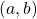stands for: greatest common divisor. You are looking for the largest factor that simultaneously divides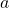and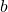evenly. If you rethink in terms of multiplication, you are finding the biggest tile size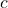that can simultaneously coverandwithout gaps:

Finds.t.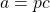and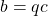where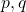are integers.

Imagine you are a lazy and cheap contractor who have unlimited tile samples of all sizes to try before making a large order:

• [Goal] you have to cover with two floors with potentially different sizesandgaplessly
• [Constraint: divisible] your customer won’t let you cut (fractional) tiles because it’s ugly
• [Constraint: common denominator] you can only order ONE tile size to take advantage of bulk discounts
• [Objective: greatest] more tiles means more work to lay it, so you want to shop for the biggest tile size that does the job.

For simplicity of the argument, we also assume the divisoris the smaller number. Ifis the bigger number, the roles will reverse in the next round because the remainder from dividing by a larger number is the dividend itself, which becomes the divisor in the next round while the old divisor (the larger number) becomes the dividend.

For relatively prime pairs, the algorithm will eventually stop with a GCD ofbecausedivides all integers.

Here’s the analog of Euclid algorithm:

• start out with 1 big tile covering the entire smaller floor• see if we can coverwithout gaps using big tiles of size• if yes, we are done., the macro-tile is your GCD (perfect-sized tile).
• if there are gaps (non-zero remainder), pick a tile-size that covers the ugly gap (the remainder becomes the divisor), then repeat the same process above over the last tile size(the divisor becomes the dividend) until there are no gaps (remainder become zero).

The algorithm is taking advantage of the fact the remainder is smaller than the last tile size (divisor) so we can rewrite the last tile size in multiples of the remainder (old gap) plus the new gap. By the time the new gap divides the last tile size, all numbers before that can be written as multiples of it (i.e. the last gap evenly divides all the numbers in the chain all the way up toand).

Let’s start with a simple case GCD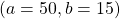that terminates early: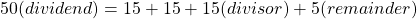We focus on the last tile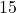and write it in terms of the old remainder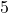: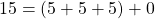Since the new remainder is, the old remainderdivides the last tile. Since we are dividingin terms of the remainder, we have

\begin{aligned}
b &= 15 \\
&= (5+5+5) \\
&= 3r \\
\\
a &= 50 \\
& = (15 + 15 + 15) + 5\\
& = 3b + r\\
&= (5+5+5) + (5+5+5) + (5+5+5) + 5\\
&= 3(3r)+r \\
& = 10r
\end{aligned}

So bothandcan be written in terms of integer multiples of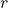right before the algorithm stops.

GCD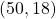takes a few more steps:

\begin{aligned}
b &= 18\\
a &= 50\\
&= (18 + 18) + 14\\
&=2b + r\\
r &= 14 \\
\\
b_1 &= r = 14\\
a_1 &= b = 18\\
&= (14) + 4\\
&= b_1 + r_1\\
r_1 &= 4\\
\\
b_2 &=r_1 = 4\\
a_2 &= b_1 = 14\\
&= (4+4+4)+2\\
&= 3b_2 + r_2\\
r_2 &= 2\\
\\
b_3 &= r_2 = 2\\
a_3 &= b_2 = 4\\
&=(2+2) + 0\\
r_3 &= 0\\

\end{aligned}

The algorithms stops atwhich means we successfully divided the last tile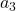with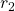(logistically stored in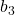) with no gaps left.(oris therefore the greatest common factor (divisor) that are shared by all the numbers involved all the way up toand.

This is the beauty of Euclid’s GCD algorithm: once the gap (remainder) is sliced small enough to evenly divide the tile (divisor) right before it, every term before it be can written in terms of integer multiples of the last number that divides the last tile without gap (no more remainder).

In our example,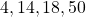can be written as a multiple of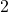, akabecause integer multiples of numbers that are already integer multiples ofcan be written in terms of a larger integer multiple of. This is why quotients do not matter in the Euclid GCD algorithm:

\begin{aligned}
b_2 &= 2r_2\\
4 &= (2+2) \\
\\
b_1 &= 3b_2 + r_2\\
&= 3(2r_2) + r_2\\
& = 7r_2\\
14 &= [(4+4+4)+2] \\
&=  [(2+2)+(2+2)+(2+2)+2] \\

\\
b &= b_1 + r_1\\
&=7r_2 + 2r_2\\
&=9r_2\\
18 &= 14 + 4 \\
&=  [(4+4+4)+2] + 4 \\
&=  [(2+2)+(2+2)+(2+2)+2]+[(2+2)] \\
\\
a &= 2b + r\\
&=2(9r_2) + 7r_2\\
&= 25r_2\\
50 & = 18 + 18 + 14 \\
&= [(2+2)+(2+2)+(2+2)+2]+[(2+2)] \cdots \\
&+ [(2+2)+(2+2)+(2+2)+2]+[(2+2)] \cdots \\
&+ [(2+2)+(2+2)+(2+2)+2]
\end {aligned}

The spirit of the algorithm is that every time we see a gap, we fill it with a small tile that’s exactly the gap size, and attempt to replace the last tile by covering it in terms of the new smaller tile (that was used to fill the gap). We are recursively slicing the last tile with the gaps produced until there are no gaps left. Once you find the GCD tile, you can replace all the bigger tiles before in terms of it with no gaps.

There’s an interesting perspective of using the Euclid GCD algorithm to solve Diophantine Equations (integer written as a linear combination of integers with integer coefficients): turns if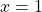in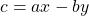is the same as writing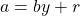. We can flip the sign of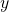by saying substituting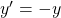.

11 total views,  1 views today

Subscribe
Notify of